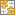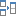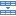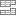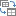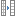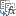# 4 ways to do a case-sensitive vlookup in Excel

The tutorial explains how to make Excel VLOOKUP case-sensitive, demonstrates a few other formulas that can look up case in Excel and points out strengths and limitations of each function.

I guess every Excel user knows what function performs a vertical lookup in Excel. Right, VLOOKUP. However, very few people are aware that Excel's VLOOKUP is case-insensitive, meaning it treats lowercase and UPPERCASE characters as identical.

Here's a quick example that demonstrates VLOOKUP's inability to distinguish case. Suppose, if you have "bill" in cell A1 and "Bill" in cell A2, the formula `=VLOOKUP("Bill", A1:A10, 2)` will catch "bill" because it comes first in the lookup array, and return a value from cell B1.

Further on in this article, I will show you a way to make VLOOKUP case-sensitive. We will also explore several other functions that can do a case-sensitive match in Excel.

We will start with fairly simple LOOKUP and SUMPRODUCT, which however have a few significant limitations. And then, we will have an in-depth look at a bit more complex INDEX / MATCH formula that works impeccably in all scenarios and on all data sets.

## A case-sensitive VLOOKUP formula

As you already know, a usual Vlookup formula is case-insensitive. However, there is a way to make Excel VLOOKUP case-sensitive. You can force it to match case by adding a helper column to your worksheet, as demonstrated in the following example.

Suppose you have "Item IDs" in column B and you want to pull the item's price and associated comment from columns C and D. The problem is that items IDs include both lowercase and uppercase chars. For example, the values in B4 (001Tvci3u) and B5 (001Tvci3U) differ in the last letter only, "u" and "U", respectively:As you can guess, a usual Vlookup formula `=VLOOKUP("001Tvci3U",\$A\$2:\$C\$7,2,FALSE)` will fetch \$90 that is associated with "001Tvci3u" because it comes before "001Tvci3U" in the lookup array. But this not what you want, right?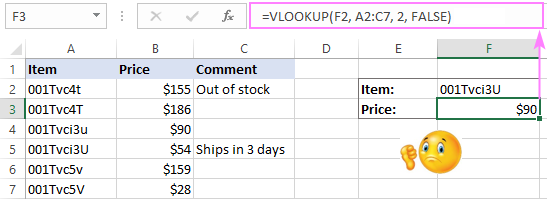To be able to perform a case-sensitive vlookup in Excel, you need to add a helper column and populate it with the below formula (where B is your lookup column):

`=CODE(MID(B2,1,1)) & CODE(MID(B2,2,1)) & CODE(MID(B2,3,1)) & CODE(MID(B2,4,1)) & CODE(MID(B2,5,1)) & CODE(MID(B2,6,1)) & CODE(MID(B2,7,1)) & CODE(MID(B2,8,1)) & IFERROR(CODE(MID(B2,9,1)),"")`

The formula parses the lookup value into individual characters, converts each char to its code (e.g. "A" is 65 and "a" is 97), and then concatenates these codes into a unique number string.

After that, you use a simple Vlookup formula that matches case:

`=VLOOKUP(\$G\$3,\$A\$2:\$C\$8,3,FALSE)`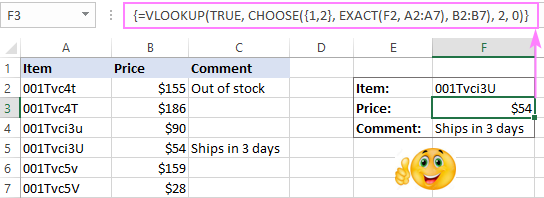The following two things are essential for the correct work of your Vlookup case-sensitive formula:

1. The helper column should be the left-most column in the lookup range (table_array argument).
2. The lookup value (lookup_value argument) shall be a "character code" rather than a real value.

#### How to use the CODE formula correctly

The CODE formula that is copied across the helper column implies that all of your lookup values have the same number of characters. If not, you need to know the min and max numbers and add as many IFERROR functions as many chars make the difference between the smallest and largest lookup values.

For example, if the smallest lookup value has 3 chars and largest - 5 chars, you use this formula:

`=CODE(MID(B2,1,1)) & CODE(MID(B2,2,1)) & CODE(MID(B2,3,1)) & IFERROR(CODE(MID(B2,3,1)),"") & IFERROR(CODE(MID(B2,4,1)),"")`

In the MID function, you specify the following arguments:

• 1st parameter (text) - this is text or a cell reference containing the characters you want to extract (B2 in our case).
• 2nd parameter (start_num) - the position of the first character you want to extract. You enter 1 in the first MID function, 2 in the second and so on.
• 3rd parameter (num_chars) - specifies the number of characters you want to return from text. Since you always want just 1, you enter "1" in all the functions.

VLOOKUP limitations: The VLOOKUP function is not the best way to do a case-sensitive lookup in Excel because, firstly, it requires adding a helper column, and secondly, it works better on homogeneous data sets, or at least when you know the exact number of symbols in your lookup values. If this is not your case, please try out other solutions that follow below.

## LOOKUP formula for case-sensitive match

Excel's LOOKUP function is very akin to VLOOKUP, however its syntax lets you match a case without adding a helper column. To do this, you have to use LOOKUP together with the EXACT function.

If we take the data from the previous example (without the helper column A with codes), the following Lookup / Exact formula will work a treat:

`=LOOKUP(TRUE,EXACT(\$A\$2:\$A\$7,\$F\$2),\$B\$2:\$B\$7)`

The formula searches through cells A2:A7 for the exact case-sensitive value in cell F2 and returns a value from column B in the same row.

As well as VLOOKUP, the LOOKUP functions works for numerical and text values equally well, and the below screenshot is a good evidence:Important! For the LOOKUP formula to work correctly, the values in your lookup column must be sorted in ascending order, i.e. from smallest to largest.

Let me briefly explain the use of the EXACT function in the above formula because this is the key point.

Excel's EXACT function compares two text values in the 1st and 2nd arguments and returns TRUE if they are exactly the same, FALSE otherwise. What is really important for us is that EXACT is case-sensitive.

Now, let's analyze how our LOOKUP / EXACT formula works: `=LOOKUP(TRUE,EXACT(\$A\$2:\$A\$7,\$F\$2),\$B\$2:\$B\$7)`

• The EXACT function checks the value of cell F2 against all the items in the range A2:A7 and if an exact case-sensitive match is found, returns TRUE, otherwise - FALSE.
• Since you've entered TRUE in the first parameter (lookup_value) of the LOOKUP function, it pulls a corresponding value from the return column (column B in this example), only when the exact case-sensitive match is found.

Hopefully, the above explanation makes sense and you understand the general idea now. If you do, you won't have any difficulties with other functions that we are going to explore next, since all of them are based on the same principle.

LOOKUP limitations: requires sorting the lookup column in ascending order.

## SUMPRODUCT - look up case of text values and return matching numbers

As you've already understood from the heading, SUMPRODUCT is yet another Excel function can do a case-sensitive lookup, but can return numeric values only. If this is not your case, you can skip directly to INDEX MATCH that provides a general solution and works on all data types.

First off, let me briefly explain the syntax of this function, it will help you better understand the case-sensitive SUMPRODUCT formula that follows below.

Excel's SUMPRODUCT multiplies components in the specified arrays, and returns the sum of the products. Its syntax is as follows:

SUMPRODUCT(array1,array2,array3, ...)

Since we want a case sensitive lookup, we use the EXACT function from the previous example as one of the multipliers:

`=SUMPRODUCT((EXACT(\$A\$2:\$A\$7,\$F\$2)*(\$B\$2:\$B\$7)))`

As you remember, the EXACT function compares the value in cell F2 against all the items in column A and if an exact case-sensitive match is found, returns TRUE, otherwise FALSE . In mathematical operations, Excel treats TRUE as "1" and FALSE as "0", so the SUMPRODUCT function multiplies the returned numbers and sums the products.

Zeros do not count, because a zero always produces "0" whatever number it is multiplied by. So, let's have a closer look at what happens when an exact match is found in column A and "1" is returned. The SUMPRODUCT function multiplies 1 by a number in column B in the same row and returns exactly this number! This is because the products of other multiplications are zeros, which do not impact the returned value in any way.

Regrettably, the SUMPRODUCT function cannot cope with text values and dates since they cannot be multiplied. In this case, you will get the #VALUE! error message like in cell F4 in the screenshot below: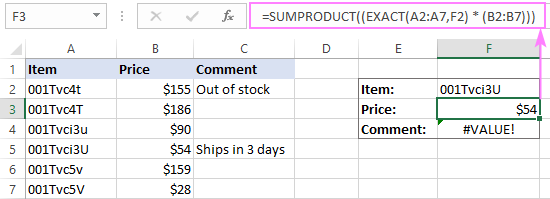SUMPRODUCT limitations: returns numeric values only.

## MATCH / INDEX - case-sensitive lookup for all data types

Finally, you are close to getting a limitation-free case-sensitive lookup formula that works on all data sets.

This example comes last of all not only because the best is saved for the last, but also because the knowledge you've gained from the previous examples may help you understand the case-sensitive MATCH / INDEX formula better.

As you probably know, the combination of INDEX and MATCH functions is used in Excel as a more flexible and powerful alternative to VLOOKUP. The following article does a good job (hopefully : ) explaining how these two functions work together - Using INDEX / MATCH instead of VLOOKUP.

I will just remind you the key points:

• The MATCH function searches for a lookup value in a specified range (lookup_array) and returns its relative position in the array.
• The relative position of the lookup value goes directly to the row_num argument of the INDEX function instructing it to return a value from that row.

For the formula to be able to recognize a case, you just need to add one more function to the classic INDEX / MATCH combination. As you can easily guess, you need the EXACT function again:

`=INDEX(\$B\$2:\$B\$7,MATCH(TRUE,EXACT(\$A\$2:\$A\$7,\$F\$2),0))`

In the above formula, the EXACT function works in the same manner as in the LOOKUP formula and produces the same result.

However, in this case we need an array formula to get it to work, so be sure to press Ctrl + Shift + Enter to complete your formula. If done correctly, the formula will get enclosed in curly braces, as shown in the screenshot below: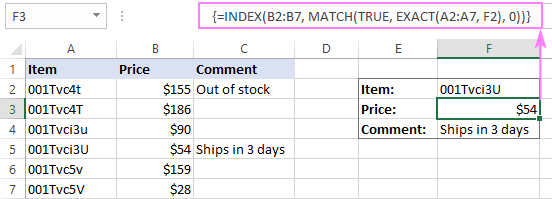#### Why INDEX / MATCH is the best solution for case-sensitive vlookup

The main advantages of using INDEX / MATCH are:

1. It does not require adding a helper column like the VLOOKUP function does.
2. It does not require sorting a lookup column like the LOOKUP function does.
3. It works with all data types - numbers, text and dates.

The above mentioned case-sensitive INDEX / MATCH formula seems perfect, right? But, in fact, it is not. Let me show you why.

Suppose, a cell in the return column corresponding to a lookup value is blank. What shall the formula return? Nothing. And now, let's see what it actually returns: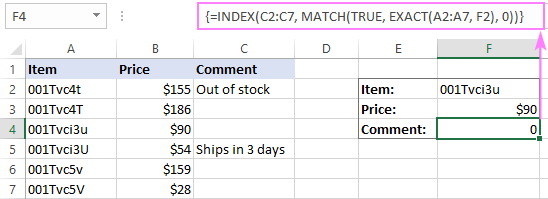Oops, the formula returns a zero! Maybe, this is no big deal if you are working solely with text values. However, if your worksheet contains numbers and some of them are real zeros, this is a problem.

In truth, all other lookup formulas (VLOOKUP, LOOKUP and SUMPRODUCT) we have discussed earlier behave in the same way. But now you want an impeccable formula, don't you?

To make the case-sensitive INDEX / MATCH formula absolutely perfect, you need to wrap it in the IF function that will check whether a return cell is blank and return nothing in this case:

`=IF(INDIRECT("B"&(1+MATCH(TRUE,EXACT(\$A\$2:\$A\$7,\$G\$2),0)))<>"",INDEX(\$B\$2:\$B\$7,MATCH(TRUE,EXACT(\$A\$2:\$A\$7,\$G\$2),0)),"")`

In the above formula:

• "B" is the return column
• "1+" is the number that turns a relative position of a cell returned by the MATCH function into a real cell address. For example, the lookup array in our MATCH function is A2:A7, meaning the relative position of cell A2 is "1", because this is the first cell in the array. But the real position of cell A2 in the column is "2", so we add 1 to compensate the difference, for the INDIRECT to return a value from the right cell.

The below screenshots demonstrate the improved case-sensitive INDEX / MATCH formula in action. I've re-written the formula for columns B:D so that the formula bar can fit within the screenshots.

The INDEX / MATCH formula returns nothing if the return cell in empty: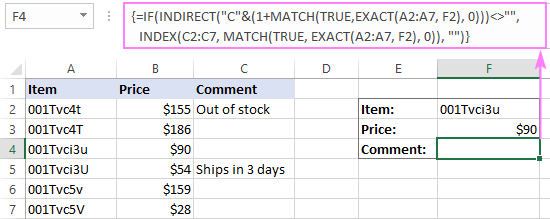The formula returns "0" if the return cell contains a zero: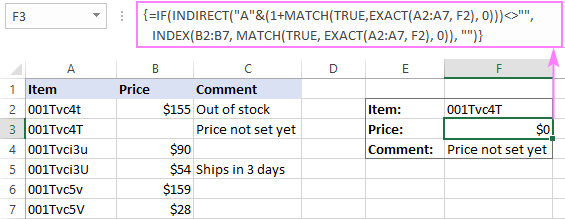If you want the INDEX / MATCH formula to display some message when a return value is blank, you can write something between the last quotes ("") in the formula, like this:

`=IF(INDIRECT("D"&(1+MATCH(TRUE,EXACT(\$B\$2:\$B\$7,\$G\$2),0)))<>"",INDEX(\$D\$2:\$D\$7,MATCH(TRUE,EXACT(\$B\$2:\$B\$7,\$G\$2),0))," There is nothing to return, sorry.")`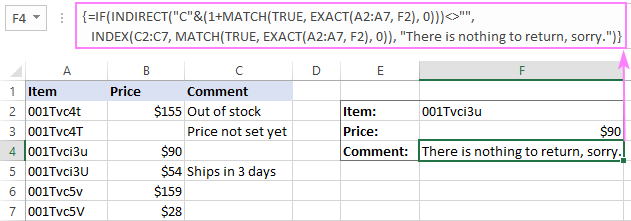That's how to lookup in Excel taking into account the text case. I thank you for reading and hope to see you on our blog next week!

Case-sensitive vlookup examples (.xlsx file)

### 27 responses to "4 ways to do a case-sensitive vlookup in Excel"

1.Tim Murphy says:

Really helpful instructions for the case-sensitive vlookup. Thanks for sharing.

2.Saif says:

Thanks a lot Svetlana!

I have been searching for a solution for quite some time now and I finally happen to stumble upon your article. This was of real help to me.

Using CODE function was really out of the box thing which is best for resolving my issue. It helped me do a Case sensitive VLOOKUP on 15 digit Salesforce.com record IDs.

The presentation was excellent as well.

Thanks again!

•Scout4u says:

Hi Saif,

I, too, need to run a Salesforce ID match, but didn't quite understand how to use that code to create a text string long enough for a 15 digit character.
May I bother you to show me your formula?

Thanks,
Lynn

3.Plan B says:

Great article but try a proof-read or two next time, particularly when describing function names. :)

•Svetlana Cheusheva (Ablebits.com Team) says:

Always do. But somehow they manage to slip by me once in a while, sorry for that.

4.Scout4u says:

Hello Svelana,

Your solution for Case Sensitive LOOKUP is not working for me. I've created a formula exactly as you have described and sorted the LOOKUP column A-Z.
For some reason the results are skipping 3 rows of data and inserting the results of the 3 row down from the exact match?
:(

5.Scout4u says:

I found another formula online that worked!
=LOOKUP(1,1/EXACT(\$A2:\$A4,\$C2),\$B2:\$B4)

This came from this blog: http://stackoverflow.com/questions/7738097/exact-case-matching-with-excel-vlookups

•Carmen Lee says:

6.Luna says:

I want to extract the duplicate list in a column. Please help what function to use. Thanks.

7.danish says:

M not able to understand vlookup

8.danish says:

Please tell me how can I apply vlookup in multiple columns at a time

9.Maurizio says:

I was searching the way to make lookup case sensitive and I found this article, the INDEX/MATCH seems interesting however I cannot make it working, in particular the function "EXACT(\$B\$2:\$B\$7,\$G\$2)" does not work with a range (\$B\$2:\$B\$7), it always compare only the first value (\$B\$2).

•Jason K says:

So true! EXACT is not working with ranges for me at all. Somehow LOOKUP understands it, but MATCH certainly is not. It always returns #N/A.

10.maryam says:

Thanks a lot.

11.Bosozoq says:

help plz can i include =upper in =VLOOKUP(A4,List!A:AG,22,)

12.Srikanth says:

Guys a very easy formula there to do this using code formula. Using code formula you can convert those case sensitive item into unique numbers.

13.Manoj Adhikari says:

I want to how is interest calculated by banks in savings and current a/C with regular deposits and withdrawals on monthly basis?

14.Dev says:

Many thanks Svetlana, worked for me, this is a common problem faced by many and I am sure this post will be of great help.

15.Dan says:

I just want to say that this article saved my life during a Salesforce migration, where many unique IDs require case sensitivity. This workaround made everything possible.

16.Mike says:

Svetlana, you are wonderful. I too was looking for a solution to using vLookup on Salesforce IDs. While there is a 15 char to 18 char conversion site that can make vLookup work, it takes extra effort. Your ...match(true,exact)... formula works much faster and simpler. Thanks!

17.Daniel Juncos says:

! (among many others) have identical returns for =code(mid(B1,1,1))&code(mid(B1,2,1))

18.Sumit Singhal says:

The first solution of helper column is really helpful particularly when all the look-up values have same number of character, as in my case.

19.mike nimri says:

I found a simple way to use VLOOKUP() + EXACT() functions for Case Sensitive validation:
Lets say in Worksheet A I have a list of Topics in Column A
Lets say in Worksheet B I have a list of students (Col A) and in column B the Topic they choose. I want to make sure they used an existing Topic with Case Sensitivity

Step 1: in Worksheet B I add a Dummy Column C with formula =VLOOKUP(B2,topics!\$A:\$A,1,FALSE) . this will get me the name of the Topic in Topic Worksheet but not case sensitive

Step2: in Worksheet B I add another Dummy Column D with formula =EXACT(B2,C2) this will compare the name I used as Topic to the name I found via Vlookup. If value if FALSE it means that there is issue with case sensitive letters (you can add conditional format to color it)

20.Brady says:

There is an unfortunate misspelling in the 2nd paragraph. I think you are trying to say "meaning it treats lowercase and UPPERCASE characters as identical." But the word "treats" is missing an "r".
Thanks for this very informative post.

•Svetlana Cheusheva (Ablebits.com Team) says:

Thank you for pointing that out! Fixed.

21.Imran Masud says:

Thanks, Svetlana!!
I am very thankful to you for your nice efforts. carry on..

22.Richard says:

Thanks Svetlana - this is great.

Can I ask please (this seems to have been asked before) - I need to do the same for Salesforce ID's, which use case to make them unique.
They are 15 characters long (always) - is it simply the case of adding "code" to your formula?
i.e =CODE(MID(D2,1,1)) & CODE(MID(D2,2,1)) & CODE(MID(D2,3,1)) & CODE(MID(D2,4,1)) & CODE(MID(D2,5,1)) & CODE(MID(D2,6,1)) & CODE(MID(D2,7,1)) & CODE(MID(D2,8,1)) & CODE(MID(D2,9,1)) & CODE(MID(D2,10,1)) & CODE(MID(D2,11,1)) & CODE(MID(D2,12,1)) & CODE(MID(D2,13,1)) & CODE(MID(D2,14,1)) & CODE(MID(D2,15,1)) & IFERROR(CODE(MID(D2,16,1)),"")

I've tried this - Excel really doesn't like it. I have 145,000 rows, and it seems to consider every single one of the new "unique" codes to be duplicates!

Ultimate Suite for Microsoft Excel